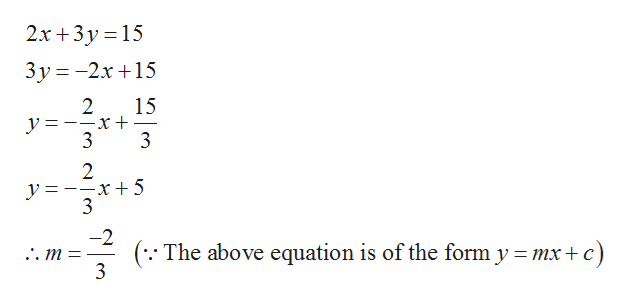# write the equation for a line, form y=mx+b for a line that is parallel to 2x+3y=15 and line passes through the point (12,-2).

Question

write the equation for a line, form y=mx+b for a line that is parallel to 2x+3y=15 and line passes through the point (12,-2).

check_circle

Step 1

It is given that the line is parallel to 2x+3y=15 and passes through...help_outlineImage Transcriptionclose2x 3y 15 3y-2x15 2 15 y 3 2 y =--x+5 3 -2 The above equation is of the form y = mx+ c) 3 . m fullscreen

### Want to see the full answer?

See Solution

#### Want to see this answer and more?

Solutions are written by subject experts who are available 24/7. Questions are typically answered within 1 hour.*

See Solution
*Response times may vary by subject and question.
Tagged in

### Equations and In-equations